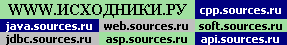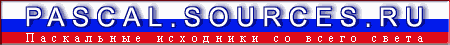Powered byНа Главную Pascal Форум Информер Страны мира
Demo Making    >>    plasma3Plasma Code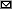Marcin Borkowski 03.02.1995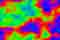Эффект плазмы. Plasma pascal sources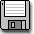1k```- ZEPAS95 --------------------------------------- English Pascal 95 - Msg : 24 of 1932 Addr Date From : Marcin Borkowski 2:480/25 03.02.95 Subj : **Plasma Code** --------------------------------------------------------------------- Hi Sean! 30 Jan 95 15:54, Sean Walker wrote to Marcin Borkowski: MB> If you wait for the retrace each time, you won't MB> flicker, but you will limit the speed to the MB> retrace speed (about 68-70) frames a second. If MB> you wanted to truly find the speed, do it without MB> the retrace wait, and you may get much faster. You may MB> already know this, in which case I am just MB> wasting space... MB>> And if you use a tripple buffering you will get no flicker and MB>> the maximu speed. SW> What the heck is triple buffering? You are using THREE different screens (no problem in x-mode). One (A) is displayed, second (B) is waiting, third (C) is being drawn. After third C is ready B is being displayed and A is being drawn. No flickering, no waiting, no problem... OK, so take a look - and learn ;-) I hope it's enough to get the idea, isn't it?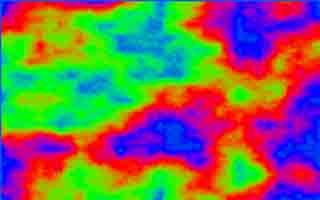Borek Please feel free to correct my English! --- GEcho 1.00 * Origin: Somewhere in Europe (2:480/25) {> Cut here. FileName= PLASMA3.PAS } { From : Marcin Borkowski 2:480/25 03.02.95} { Subj : **Plasma Code**} {\$A+,B-,D+,E+,F-,G+,I-,L+,N-,O-,P-,Q-,R-,S-,T-,V+,X+,Y+} {\$M 16384,0,655360} uses crt; var i : integer; licznik : byte; paleta : array[0..767]of byte; screen : array[0..63999]of byte absolute \$A000:0; { This is necessaery for drawing plasma. Don't mind. It is the same piece of code I use in voxel space code posted here for several times, not necessarilly by me. } function ncol(mc,n,dvd : integer): integer; var loc : integer; begin loc:=(mc+n-random(2*n)) div dvd; ncol:=loc; if loc>250 then ncol:=250; if loc<5 then ncol:=5 end; procedure plasma(x1,y1,x2,y2 : word); var xn,yn,dxy,p1,p2,p3,p4 : word; begin if (x2-x1<2) and (y2-y1<2) then EXIT; p1:=screen[320*y1+x1]; p2:=screen[320*y2+x1]; p3:=screen[320*y1+x2]; p4:=screen[320*y2+x2]; xn:=(x2+x1) shr 1; yn:=(y2+y1) shr 1; dxy:=5*(x2-x1+y2-y1) div 3; if screen[320*y1+xn]=0 then screen[320*y1+xn]:=ncol(p1+p3,dxy,2); if screen[320*yn+x1]=0 then screen[320*yn+x1]:=ncol(p1+p2,dxy,2); if screen[320*yn+x2]=0 then screen[320*yn+x2]:=ncol(p3+p4,dxy,2); if screen[320*y2+xn]=0 then screen[320*y2+xn]:=ncol(p2+p4,dxy,2); screen[320*yn+xn]:=ncol(p1+p2+p3+p4,dxy,4); plasma(x1,y1,xn,yn); plasma(xn,y1,x2,yn); plasma(x1,yn,xn,y2); plasma(xn,yn,x2,y2) end; begin asm mov ax,13h int 10h end; { Generating palette RGBs } for i:=1 to 170 do paleta[3*i]:=round(63*sin(i/170*pi)); for i:=1 to 170 do paleta[3*i+256]:=round(63*sin(i/170*pi)); for i:=1 to 170 do paleta[(3*i+512) mod 768]:=round(63*sin(i/170*pi)); plasma(1,1,319,199); { Licznik - it means 'counter' in Polish. } licznik:=0; repeat { Wait for retrace. } repeat until (port[\$03DA] and 8)=0; repeat until (port[\$03DA] and 8)=8; { Changing palette - we start with color number licznik } port[\$3C8]:=licznik; { Three outsb are copying whole RGB to VGA register. After those three instructions value in port \$3C8 is incremented. Here I'm redefining whole palette, but there is no problem in changing only one color. } asm mov si,offset paleta mov cx,768 mov dx,\$3C9 rep outsb end; inc(licznik); until keypressed; asm mov ax,3h int 10h end; end.```